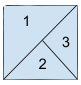New update is available. Click here to update.

# Regions Cut By Slashes

Last Updated: 19 Mar, 2021
Difficulty: Moderate

## PROBLEM STATEMENT

#### This time our favorite Ninja wants to buy a house. But they are unable to count the number of rooms in the house. So they need your help to count the number of rooms.

##### Note:
``````A house is represented by a matrix where “\” and “/” represent a wall and blank space is empty space. We will consider two rooms different if we will be unable to reach another room.
``````

#### Your task is to return the number of rooms. For a better explanation, see the example.

##### Example:
``````Input:
[
“ /”
“/\”
]

Output: 3

Explanation: 2 X 2 house is shown below:
``````##### Input Format:
``````The first line of input contains an integer 'T' representing the number of test cases.

The first line of each test case contains the ‘N’ number of strings.

The next ‘N’ lines of each test case contain a single string of length ‘N’.
``````
##### Output Format:
``````For each test case, return the number of the separate rooms.

The output of each test case will be printed in a separate line.
``````
##### Note:
``````You do not need to print anything, it has already been taken care of. Just implement the given function.
``````
##### Constraints:
``````1 <= T <= 5
2 <= N <= 30

Time limit: 1 sec
``````